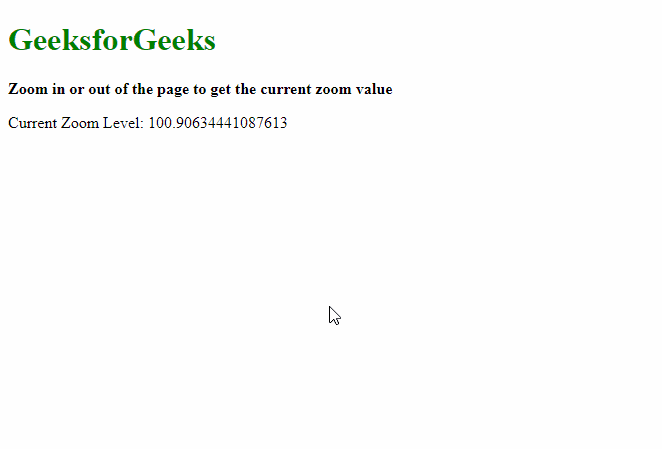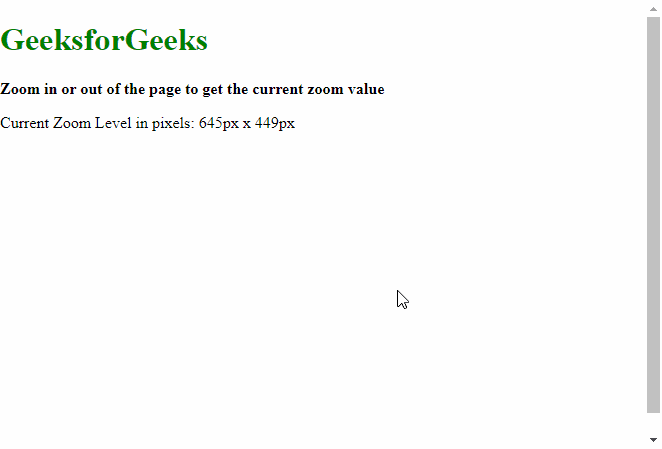# How to detect page zoom level in all modern browsers using JavaScript ?

• Last Updated : 15 Jul, 2020

In this article, we will discuss how the current amount of zoom can be found on a webpage.

Method 1: Using outerWidth and innerWidth Property: It is easier to detect the zoom level in webkit browsers like Chrome and Microsoft Edge. This method uses the outerWidth and innerWidthproperties, which are the inbuilt function of JavaScript. The outerWidth is first subtracted by 10, to account for the scrollbar and then divided with the innerWidth to get the zoom level.

Hey geek! The constant emerging technologies in the world of web development always keeps the excitement for this subject through the roof. But before you tackle the big projects, we suggest you start by learning the basics. Kickstart your web development journey by learning JS concepts with our JavaScript Course. Now at it's lowest price ever!

Note: The amount of zoom may not be exactly the amount of zoom shown by the browser. This can be solved by rounding off the value.

Syntax:

`let zoom = (( window.outerWidth - 10 ) / window.innerWidth) * 100;`

Example:

## HTML

 ```<``html` `lang``=``"en"``>`` ` `<``body``>``    ``<``h1` `style``=``"color: green"``>``        ``GeeksforGeeks``    ```` ` `    ``<``b``>``        ``Zoom in or out of the page to``        ``get the current zoom value``    ```` ` `    ``<``p``>Current Zoom Level:``        ``<``span` `class``=``"output"``>``        ````    ```` ` `    ``<``script``>``        ``window.addEventListener(``            ``"resize", getSizes, false);`` ` `        ``let out = document.querySelector(".output");`` ` `        ``function getSizes() {``            ``let zoom = ((window.outerWidth - 10)``                ``/ window.innerWidth) * 100;``                 ` `            ``out.textContent = zoom;``        ``}``    `````` ` ``

Output:Method 2: Using clientWidth and clientHeight Property: As finding the amount of zoom is not possible in several browsers, the dimensions of the website can be found out and the operation can be done using these dimensions of the page. This method uses the clientWidth and clientHeigh properties, which are the inbuilt function of JavaScript.

Syntax:

`let zoom = body.clientWidth + "px x " + body.clientHeight + "px";`

Example:

## HTML

 ```<``html` `lang``=``"en"``>`` ` `<``head``>``    ``<``style``>``        ``/* Set the body to occupy the``           ``whole browser window``           ``when there is less content */``        ``body {``            ``height: 100vh;``            ``margin: 0;``        ``}``    `````` ` `<``body``>``    ``<``h1` `style``=``"color: green"``>``        ``GeeksforGeeks``    ```` ` `    ``<``b``>``        ``Zoom in or out of the page to``        ``get the current zoom value``    ```` ` `    ``<``p``>``        ``Current Zoom Level in pixels:``        ``<``span` `class``=``"output"``>``        ````    ```` ` `    ``<``script``>``        ``window.addEventListener(``                ``"resize", getSizes, false);``                 ` `        ``let out = document.querySelector(".output");`` ` `        ``function getSizes() {``            ``let body = document.body;``            ``let zoom = body.clientWidth + "px x " +``                ``body.clientHeight + "px";``            ``out.textContent = zoom;``        ``}``    `````` ` ``

Output:My Personal Notes arrow_drop_up